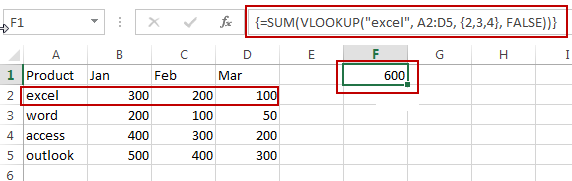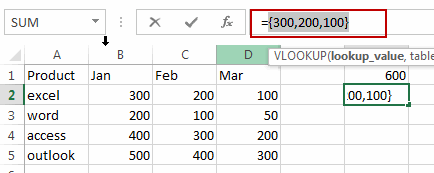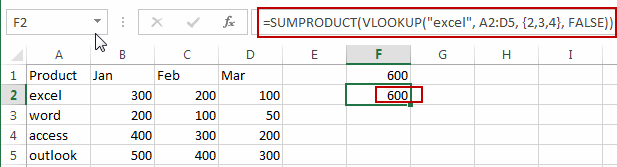# How to Vlookup to Return the SUM of Two or More Columns in Excel

This post will guide you how to lookup a value and then return the sum of two or more related columns in Excel 2013/2016. How do I Use VLOOKUP function and SUM function to lookup a specific value and return the sum of all matches in columns in Excel.

## Vlookup to Return the Sum of Two or More Columns

Assuming that you have a list of data in range A1:D5, in which contains sales data for three months. And you want to look up a product named “excel” in this range, and then return the sum of three months or columns. How to do it. You can use the VLOOKUP function to look up values in the given range, but the problem is that this function returns only one related sales value.  If you want the VLOOKUP function to return the values from the two or more columns, you need to put column numbers that you want returned into VLOOKUP function as its third argument.  Then the VLOOKUP function will also return an array values. Last, you can use the sum function to calculate the total value of the returned array values from VLOOKUP function.

So you can write down an Excel Array formula based on the VLOOKUP function and the SUM function as below:

`=SUM(VLOOKUP("excel", A2:D5, {2,3,4}, FALSE))`

Type this formula into a blank cell and press Ctrl + Shift +Enter keys on your keyboard to change it as array formula. You would notice that the total sales value of product “excel” in three months has been calculated.Note:

• “excel” is the lookup value
• A2:D5 is the lookup range
• {2,3,4} are the column numbers that have sales values you want to return, it should be enclosed in curly braces so that to create the array of values.

Let’s see how this formula works:

= VLOOKUP(“excel”, \$A\$2:\$D\$5, {2,3,4}, FALSE)

This formula returns an array result that contain three sales values of product “excel”.You can also use another formula based on the SUMPRODUCT and VLOOKUP functions to achieve the same result. Like this:

`=SUMPRODUCT(VLOOKUP("excel", A2:D5, {2,3,4}, FALSE))`

Type this formula into a blank cell and press Enter key.The SUMPRODUCT function will return the sum of the array elements returned by the VLOOKUP Function.

### Related Functions

• Excel VLOOKUP function
The Excel VLOOKUP function lookup a value in the first column of the table and return the value in the same row based on index_num position.The syntax of the VLOOKUP function is as below:= VLOOKUP (lookup_value, table_array, column_index_num,[range_lookup])….
• Excel SUM function
The Excel SUM function will adds all numbers in a range of cells and returns the sum of these values. You can add individual values, cell references or ranges in excel.The syntax of the SUM function is as below:= SUM(number1,[number2],…)…
• Excel SUMPRODUCT function
The Excel SUMPRODUCT function multiplies corresponding components in the given one or more arrays or ranges, and returns the sum of those products.The syntax of the SUMPRODUCT function is as below:= SUMPRODUCT (array1,[array2],…)…